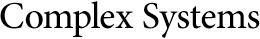## No Polynomial Bound for the Period of Neuronal Automata with Inhibitory Memory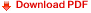René Ndoundam
Département d'Informatique,
Faculté des Sciences,
Université de Yaoundé I,
B.P 812 Yaoundé, Cameroun

Martín Matamala
We study the sequences generated by a neuronal equation with memory of the form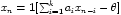, where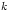is the size of the memory. We show that in the case where all the parameters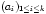are negative real numbers, there exists a neuronal equation of memory lengththat generates a sequence of period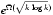. This result shows that in the case where all weighting coefficients are negative, the neuronal recurrence equation exhibits a complex behavior.